## Classification

#### Classification

Direction: In each problem, out of the five figures marked (a), (b), (c), (d) and (e), four are similar in a certain manner. However one figure is not like the other four. Choose the figure which is different from the rest.

1. Choose the figure which is different from the rest.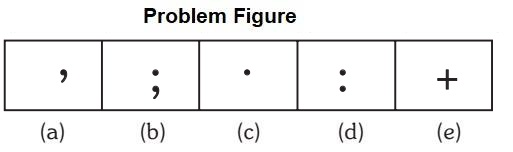1. only one symbol is mathematical.

##### Correct Option: E

Option (e) is mathematical symbol.

1. Choose the figure which is different from the rest.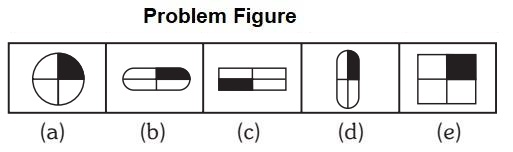1. The shaded region is on top right region.

##### Correct Option: C

The shaded region is present on the left bottom region, where as rest all has on top right region.

1. Choose the figure which is different from the rest.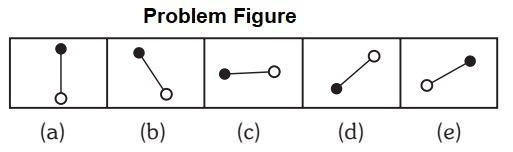1. All rotates in anti clock wise direction except one.

##### Correct Option: E

All rotates in anti clock wise direction except option (e).

1. Choose the figure which is different from the rest.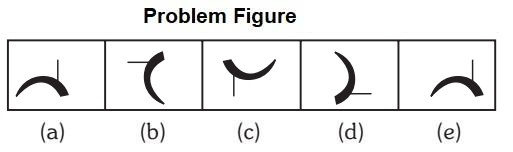1. The line segment present thick side of the arc. Whereas, answer figure has line segment on the thin side of the arc.

##### Correct Option: D

The line segment present thick side of the arc. Whereas, answer figure has line segment on the thin side of the arc.

1. Choose the figure which is different from the rest.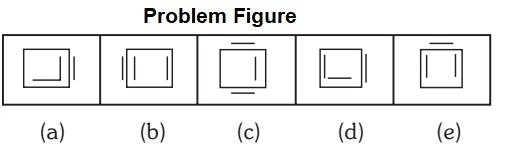1. In all other figure, the square has two line segments inside and one line segment outside.

##### Correct Option: C

In all other figure, the square has two line segments inside and one line segment outside.